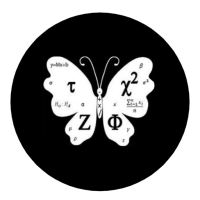## Welcome to Moore Statistics Consulting LLCIf you are taking a basic statistics course, there are certain themes that pop-up. Some of the concepts that your teacher presents to you are intuitive. If you have two of something and you want the average then you divide by two. If you are looking for the range of a set of values, your gut tells you that ‘range’ signifies the distance between the smallest and largest value. The term ‘standard deviation’ sounds like what? You want to know the standard distance from the mean. You are standardizing that distance in terms of your sample. Now there are some terms that do not come as easily and you must LEARN a new SKILL. Namely, I am referring to the Z table. In particular, I am speaking of the following scenario,

Scenario: The probability X is between two numbers.

Now if you know your z-table, you understand that it can only give you the area under the curve to the left of your z value. So a Z value of ‘0’ refers to the area to the left of zero. If you want the probability of the area to the right of zero, subtract it from 1. Why ‘1’? Because the total area under the curve equals the sum of all the probabilities. What the heck am I talking about? I am speaking of the likelihood or probability that an event will occur. In terms of a population, each member has a chance of showing up with a particular probability associated with it….but I digress…

…back to the scenario…let’s make it visual. So say you see this,

P(3<x<5): what is the probability between 3 and 5

Worked out: P(x<5) -P(x>35)= P(x<5)-( 1-P(x<3))

Whenever you see greater than a number or z value as a probability- subtract ‘1’ (one).

If you enjoyed this blog there are more to follow.-Amy